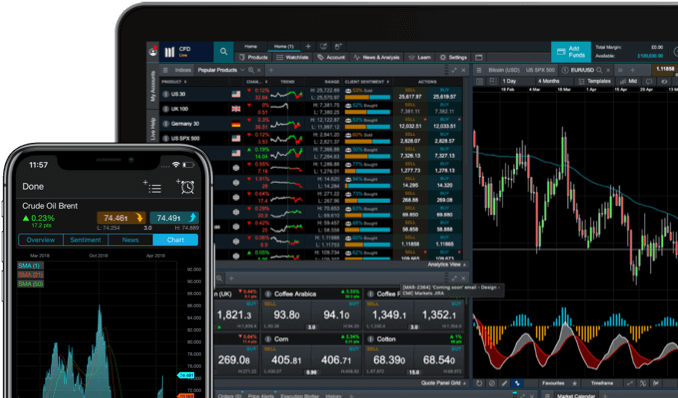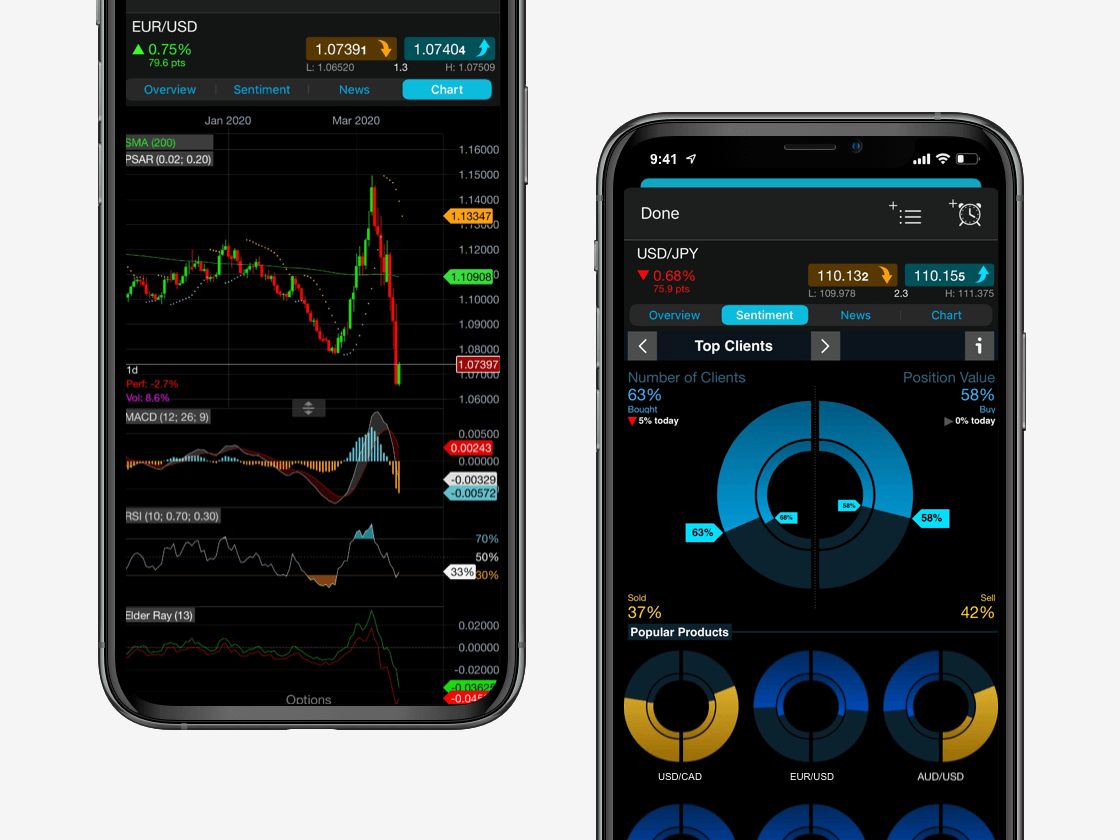# How Much Is 1 Pip Worth in Forex Trading

In trading, a ‘pip’ is a very small price movement. The term is short for ‘percentage in point’. A pip is essentially the smallest move that a currency could make in the forex market​ and it is an important unit of measurement in currency trading.

Traders use pips to measure price movements in currencies. Determining the number of pips in a certain price movement is a straightforward process, although it depends on the forex pair being traded.

11,500
instruments.## What are ‘pips’ in forex trading?

In
forex trading​, the smallest price change is the last decimal point. Given that most major currency pairs​, such as those involving USD, EUR and GBP, are priced to four decimal places, a pip in this scenario is a price movement of 0.0001. For example, if GBP/USD moved from 1.4000 to 1.4001, it has moved by one pip. Comparatively, currency pairs using the Japanese yen (JPY) are only quoted to two decimal places. In this case, a pip is a price movement of 0.01. For instance, if GBP/JPY moved from 150.00 to 150.05, it has moved by five pips.

You can trade on the forex market through financial instruments such as spread betting​ and trading CFDs​ (contracts for difference). This involves opening positions based on the prediction that one currency will strengthen against another. For example, for every pip or point that a currency’s value varies, this will result in profits or losses for the trader, depending on the direction that the market heads.

## Pips and pipettes

To view an even tighter spread, currency pairs can be given in fractional pips, or ‘pipettes’, where the decimal place is at 5 places, or 3 places if dealing JPY. A pipette is therefore equal to one tenth of a pip.

EUR/USD example:

EUR/USD = 1.60731

EUR/USD = 1.60731 – 0.0003 is the pip

EUR/USD = 1.60731
– 0.00001 is the pipette

The fourth decimal place is the pip, and the fifth decimal place is the pipette.## How to use pips in forex trading

If a trader enters a long position on GBP/USD at 1.5000 and it moves to 1.5040, the price has moved 40 pips in the trader’s favour, potentially leading to a profit if the trade is closed. On the other hand, if the trader goes long on GBP/USD at 1.5000 and the exchange rate falls to 1.4960, the price has moved 40 pips against the trader, potentially leading to a loss on the trade if it is closed.

Similarly, if a trader goes long on GBP/JPY at 145.00 and it moves to 145.75, the price has moved 75 pips in the trader’s favour. If the exchange rate goes against the trader, and GBP/JPY falls to 144.25, the price would have moved 75 pips against the trader.

As well as measuring price movements and profits and losses, pips are also useful for managing risk in forex trading and for calculating the appropriate amount of leverage​ to use. For example, a trader can use a stop-loss order​ to set the maximum amount he is willing to lose in terms of pips on a trade. Having a stop-loss in place will help to limit losses if the currency pair were to move in the wrong direction.

## Forex position size calculator

Pips can be used for the calculation of position size. If a trader’s combined position sizes are too large and they experience a number of losses, their capital could be wiped out. Therefore, trading with an appropriate position size is essential.

There are several steps involved in calculating position size:

1. A trader must determine the amount of capital they are willing to risk per trade. If this is 1% per trade, they could make a minimum of 100 trades before their capital is wiped out. If the trader’s account has a balance of \$5,000 and they are willing to risk 1% per trade, this equates to \$50 per trade.
2. Traders can determine a stop-loss in pips. For example, if a trader goes long on EUR/USD at 1.3600, they could place a stop-loss at 1.3550. This stop-loss equates to 50 pips.
3. The last step depends on what lot size is being traded. A standard lot refers to 100,000 units of base currency and equates to \$10 per pip movement. A mini lot is 10,000 units of base currency and equates to \$1 per pip movement. A micro lot is 1,000 units of base currency and equates to \$0.10 per pip movement.

If the trader risks 1% of his \$5,000 balance per trade for a micro lot (\$0.10 per pip movement), the position size would be \$50 / (50 pips x \$0.10) = 10. Therefore, the trader’s position size would be 10 micro lots.

## Pip value calculator

How much profit or loss a pip of movement produces is dependent on the value of each pip. In order to learn how to work out pip value, we need to know the following three things: the currency pair being traded, the trade amount, and the spot price.

### Pip value formula

The formula to calculate the value of a pip for a four-decimal currency pair is:

Pip value = (0.0001 x trade amount) / spot price## How to calculate pips

Example 1:

The value of USD/CAD rises to 1.0568. In this instance, one pip is a movement of 0.0001, so the trader has made a profit of 20 pips (1.0568 – 1.0548 = 0.0020 which is the equivalent of 20 pips).

The pip value in USD is (0.0001 x 100,000) / 1.0568 = \$9.46

To calculate the profit or loss on the trade, we multiply the number of pips gained by the value of each pip.

In this example, the trader made a profit of 20 x \$9.46 = \$189.20.

Example 2:

The value of USD/CAD falls to 1.0540. In this instance, one pip is a movement of 0.0001, so the trader has made a loss of 30 pips (1.0570 – 1.0540 = 0.0030 which is the equivalent of 30 pips).

The pip value in USD is (0.0001 x 10,000) / 1.0540 = \$0.94

In this example, the trader made a loss of 30 x \$0.94 = \$28.20.

### Pip value indicator on MT4

Pip values can be difficult and take time to calculate, while some traders would rather be focusing on perfecting their forex trading strategy​. This is why they have developed a pip value indicator for MetaTrader 4​, an internationally recognised trading platform that we host via our own platform. A wide range of MT4 indicators are available to download separately to your account.

Forex pips can be calculated using the formula above and displayed on our own trading platform​, Next Generation, in the form of forex price charts and graphs. These can be customised with our drawing tools. We have a wide range of technical indicators to help you with your forex trading strategy.

## What causes pip values to change?

The base value of a trader’s account will determine the pip value of many different currency pairs. For a USD-denominated account, which is common for the most traded currency pairs​, if the currency pair has USD as the second (quote) currency, the pip value will always be \$10 on a standard lot, \$1 on a mini lot and \$0.10 on a micro lot.

Pip values would only change if USD was either the first (base) currency in the currency pair, or not involved in the pair, and if the value of USD moved significantly by more than 10% in either direction.